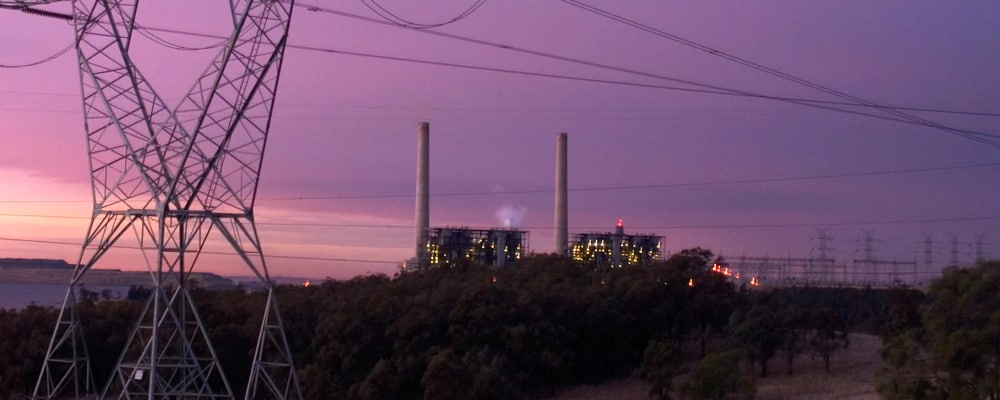There are a number of different ways of thinking about electricity which is generated from a power station. As a result, there are many different terms to describe these different generation numbers, and all are measures in the energy units kWh.

## Gross generation

At a very high level a power station is comprised of a generator which generates the electricity. The amount of electricity which is generated at this point we call the Gross Actual Generation (GAAG). This is the largest number for generation.

## Net generation

In the power station after the electricity is generated, some of the electricity is used onsite, for example in lighting and other electrical equipment. These are called auxiliary loads.

As the electricity leaves the power station it passes through a meter. This meter measures the amount of electricity leaving or coming into the power station.

## What we report

Electricity leaving the power station which is equal to the GAAG minus the auxiliary loads is called Net Actual Generation excluding imports (NAAGxi). This number is reportable under the National Greenhouse and Energy Reporting (NGER) Act.

Net Actual Generation excluding imports (NAAGxi) = Gross Actual Generation (GAAG) - Auxiliary Loads

Electricity coming into the power station through the meter is called imported electricity, and is used to run auxiliary loads when the generator is not running.

## What we supply to the grid

If we take the NAAGxi and subtract the imported electricity we are left with what is called the Net Actual Generation (NAAG). This is the figure used primarily by the energy businesses when they consider their contribution to supplying the grid, and is sometimes also called Net Sent Out generation.

Net Actual Generation (NAAG) = Gross Actual Generation (GAAG) - Auxiliary Loads - Imported Electricity

Once the electricity passes from the station onto the network AGL sells this on the wholesale market. To determine the amount paid to AGL the Australian Energy Market Operator (AEMO) must determine the amount of electricity sold. AEMO uses the NAAG number as a starting point rather than paying AGL for NAAGxi and then charging AGL for the imported electricity. AEMO adjusts the amount of electricity depending on how far away from the distribution node the station. As there are losses in transporting electricity over long distances AEMO applies a loss factor to the NAAG, this is called node generation.